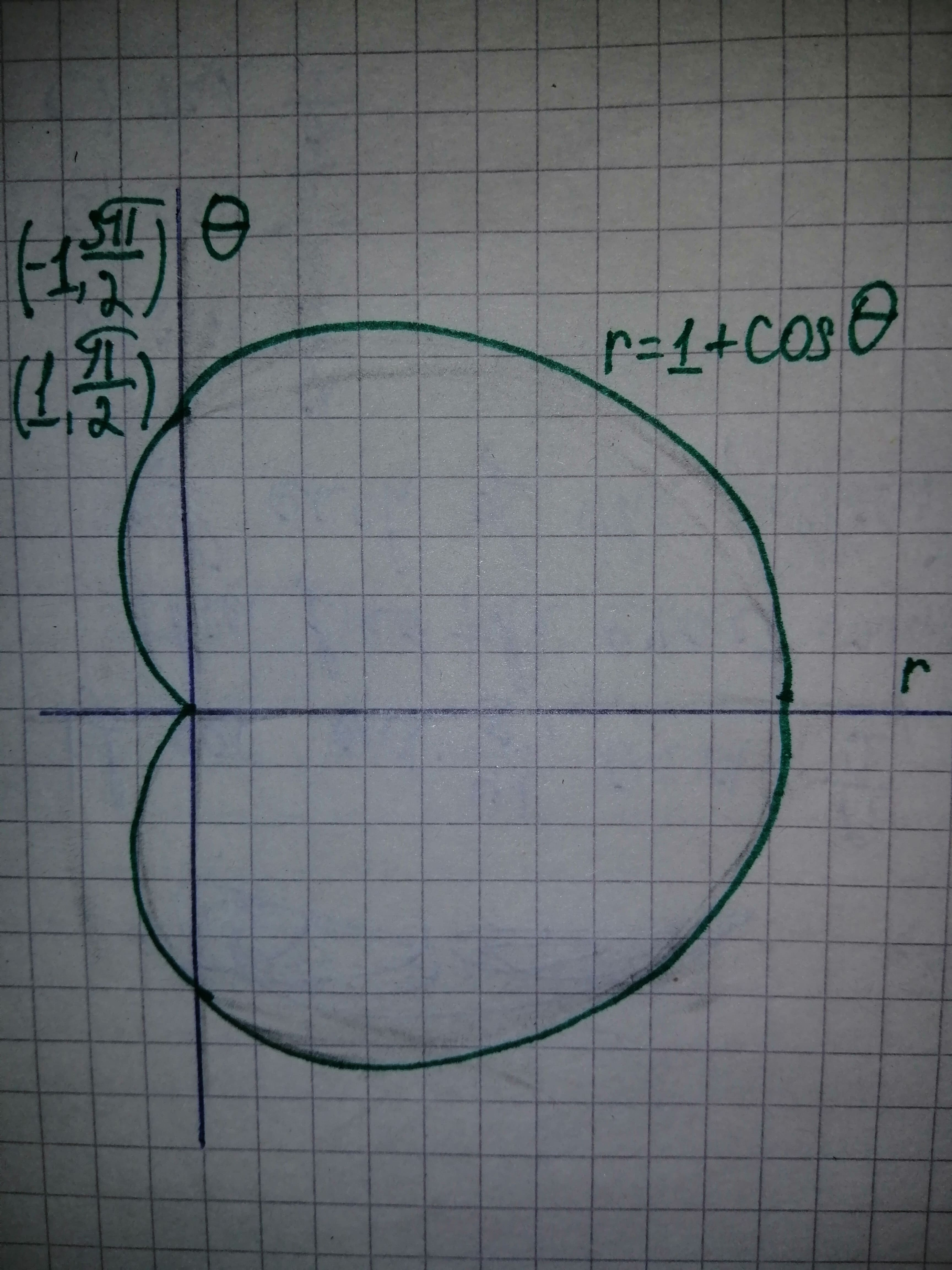# Given Vallias' value for int_{0}^{sqrt{a}} x^{2}dx, calculate his value for int_{0}^{a} sqrt{x}dx, using graph.ediculeN 2020-11-12 Answered
Given Vallias' value for ${\int }_{0}^{\sqrt{a}}{x}^{2}dx$,
calculate his value for ${\int }_{0}^{a}\sqrt{x}dx,$ using graph.
You can still ask an expert for help

• Questions are typically answered in as fast as 30 minutes

Solve your problem for the price of one coffee

• Math expert for every subject
• Pay only if we can solve itoppturf

Step 1
Let's look at this picture and look at the regionsStep 2
From the above figure, it is observed that length and breadth of the rectangle are a and square root of a. the area of rectangle can be given as follows.
Area of the $={\int }_{0}^{\sqrt{a}}{x}^{2}dx+{\int }_{0}^{a}\sqrt{x}dx$
$length×breadth={\int }_{0}^{\sqrt{a}}{x}^{2}dx+{\int }_{0}^{a}\sqrt{x}dx$
$a×\sqrt{a}={\int }_{0}^{\sqrt{a}}{x}^{2}dx+{\int }_{0}^{a}\sqrt{x}dx$
${\int }_{0}^{a}\sqrt{x}dx=a\sqrt{a}-{\int }_{0}^{\sqrt{a}}{x}^{2}dx$
Thus, for given Wallis's value for ${\int }_{0}^{\sqrt{a}}{x}^{2}dx$, it be obtained that
${\int }_{0}^{a}\sqrt{x}dx=a\sqrt{a}-{\int }_{0}^{\sqrt{a}}{x}^{2}dx$